# Excel YIELD FunctionArticle byExcelMojo Team## What Is YIELD Function In Excel?

The YIELD function in Excel belongs to the category of Financial function. It determines the yield on security, paying periodic interest. Users can apply the Excel YIELD function for evaluating a bond yield, which helps calculate the income generated in a year as the potential return.

For example, the table below contains the inputs required to determine a security’s yield (in percentage).

Suppose the requirement is to show the yield value in cell B12. Then considering the Excel YIELD function definition, applying the YIELD() in the target cell can help us achieve the required data.

In the above Excel YIELD function example, the function returns the yield value as a decimal number, 0.04877, in the target cell B12. But once we set the cell B12 data format as Percentage, the decimal value converts into a percentage value, 4.88%.

###### Key Takeaways
• The Excel YIELD function evaluates the yield on a security that pays periodic interest. The function returns a decimal value. We can set the target cell data format, containing the YIELD(), as Percentage to get the required yield as a percentage value.
• Users can utilize the YIELD() to determine a bond yield and evaluate the annual income generated.
• The YIELD() accepts six mandatory arguments, settlement, maturity, rate, pr, redemption, and frequency, and one optional argument, basis, to calculate the yield.
• The coupon payment frequency can be annual, semi-annual, or quarterly. On the other hand, we can supply five types of day count basis to the YIELD().

### YIELD() Excel Formula

The Excel YIELD formula is:

where,

• settlement: It is the security’s settlement date, falling after the issue date. And that is when the security gets traded to the buyer.
• maturity: It is the security’s maturity date, i.e., the date when the security expires.
• rate: The annual coupon rate of the security.
• pr: The cost of the security per \$100 face value.
• redemption: The redemption amount of the security per \$100 face value.
• frequency: The count of coupon payments per year.
• basis: It indicates the day count basis type used by the security.

The first six arguments in the Excel YIELD formula are mandatory. On the other hand, the last argument is optional, and the function considers its default value as 0.

And the following tables show the frequency and basis argument values we can use when applying the Excel YIELD function.

Furthermore, below are a few critical aspects that we must consider to avoid all conditions of the Excel YIELD function not working.

• Ensure to enter the date values using the DATE Excel function to avoid entering the date as a text value. And we can supply the date arguments to the Excel YIELD function as cell references to dates or functions or formulas returning date values.
• The arguments, frequency, and basis get truncated to integers. And suppose the arguments settlement or maturity are serial numbers (date equivalent values), but not integer values. Then, they also get truncated.
• If settlement or maturity is an invalid date or any of the supplied arguments are non-numeric, the YIELD() returns the #VALUE! error.
• The YIELD() return value will be the #NUM! error in the following conditions:
• settlement >= maturity
• rate < 0
• pr <= 0 or redemption <= 0
• frequency is not 1, 2, or 4
• basis is not 0, 1, 2, 3, or 4

### How To Use YIELD Excel Function?

The steps to apply the Excel YIELD function are:

1. First, ensure the inputs for determining a security’s yield are accurate and in the required data formats.
2. Then, select the target cell and enter the Excel YIELD function.
3. Press Enter to view the required security yield.
4. Finally, select the target cell and set the data format as Percentage using the Number Format option in the Home tab to view the calculated security yield as a percentage.

Check out the following example to understand the Excel YIELD function definition and the steps to use the YIELD().

The below table contains information about the security scheme for which we need to determine the yield.

And suppose we require to show the yield value in cell C13. Then here is how we can apply the YIELD() in the target cell and achieve the desired result.

1. When we check the input data, we find that the first two arguments of the YIELD() are dates, and the third argument is a percentage value. On the other hand, the fourth and fifth arguments are currency values, and the last two are numbers. So, all the arguments are in the required data formats, thus avoiding the condition of the Excel YIELD function not working due to incorrect data formats.

2. Select the target cell C13, enter the following YIELD(), and press Enter.

=YIELD(D5,D6,D7,D8,D9,D10,D11)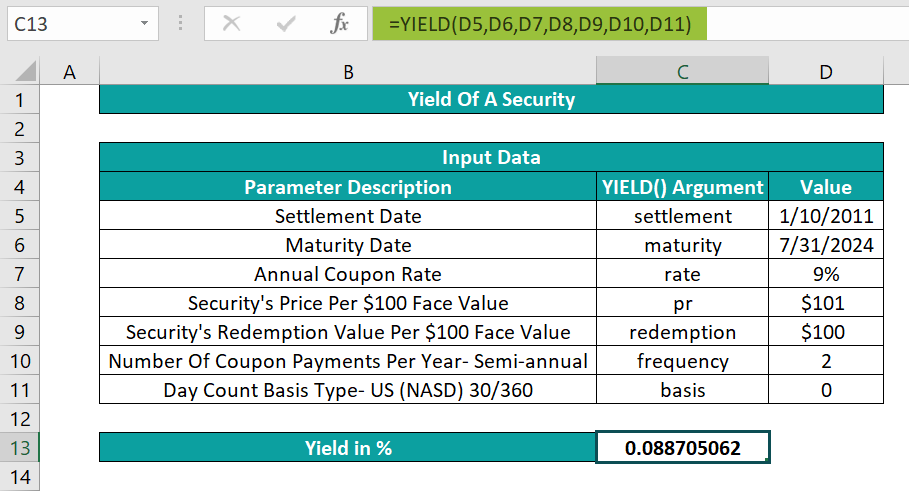In this Excel YIELD function example, the number of coupon payments per year is semi-annual or half-yearly. And hence the frequency argument value is 2. And as the day count basis type is the default value, the basis argument value is 0.

Thus, based on the supplied argument values, the YIELD() returns the required security yield value as 0.0887.

We can also provide the argument values directly to the YIELD() to achieve the above output.

Ideally, the suggestion is to supply the cell reference to the date values. But when providing the argument values directly, it is best to use the DATE() inside the YIELD() to avoid entering invalid dates.

Once we enter the formula in the target cell C13 until the argument redemption, separated by commas, we will get a drop-down list. We can use it to enter the frequency argument value by double-clicking the required option. Then, after we provide the frequency argument value and enter a comma, we will see another drop-down list to supply the basis argument value. Double-click on the required day count basis type, and we can finally close the parenthesis and press Enter to execute the YIELD().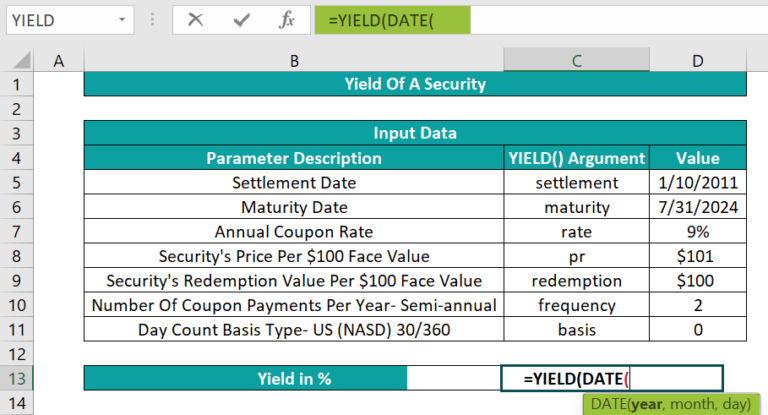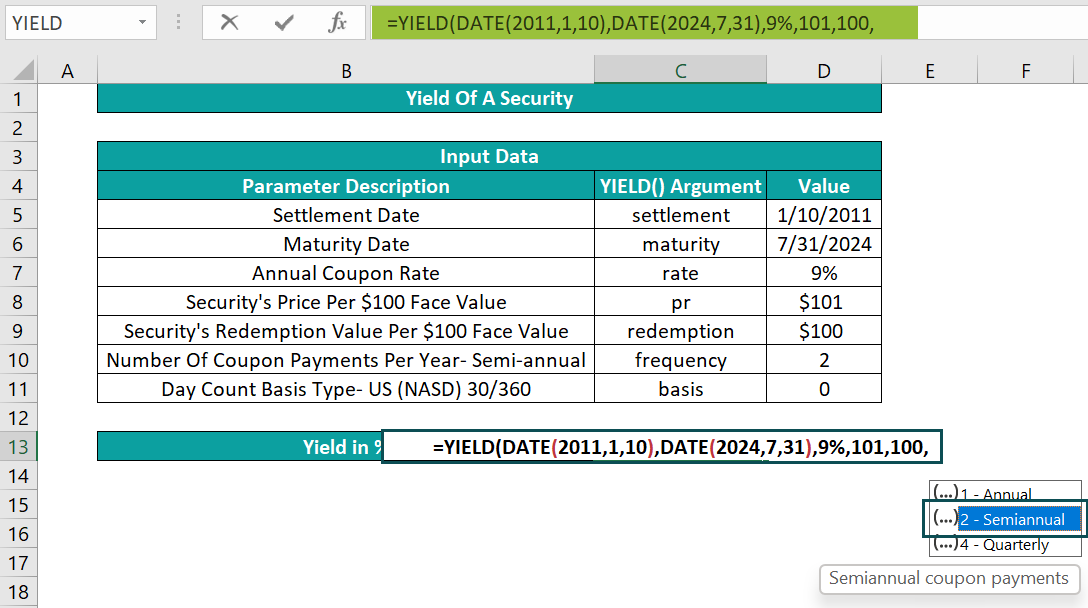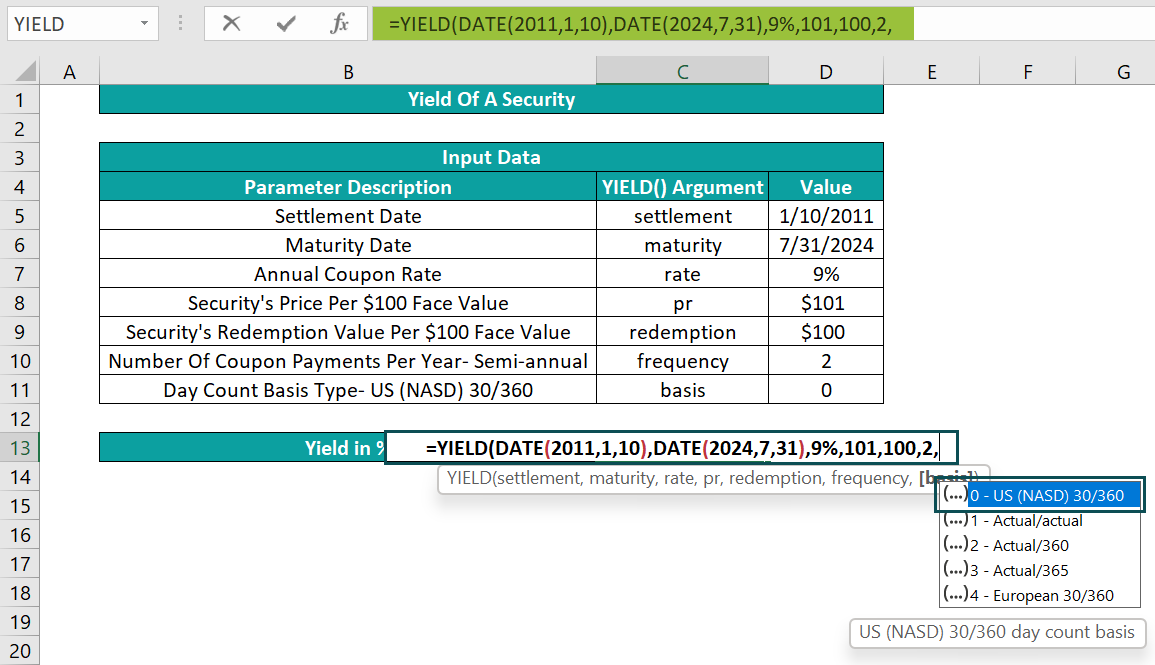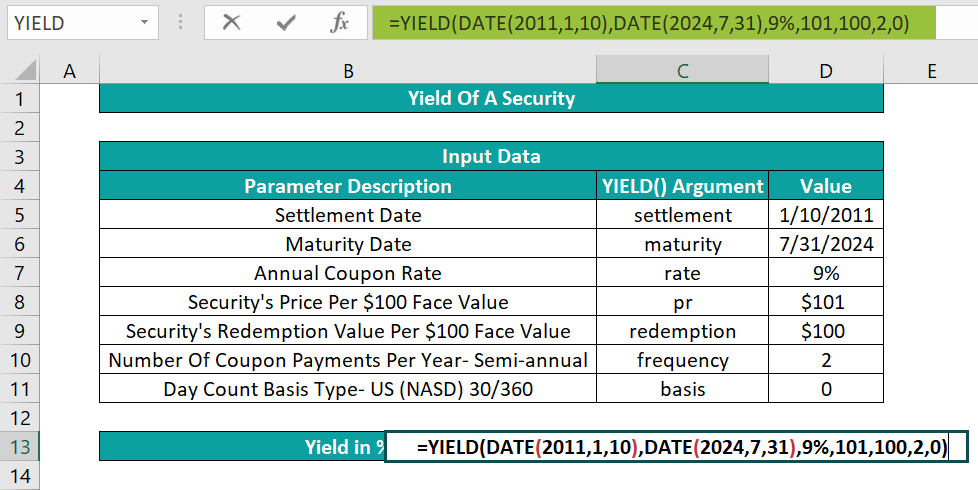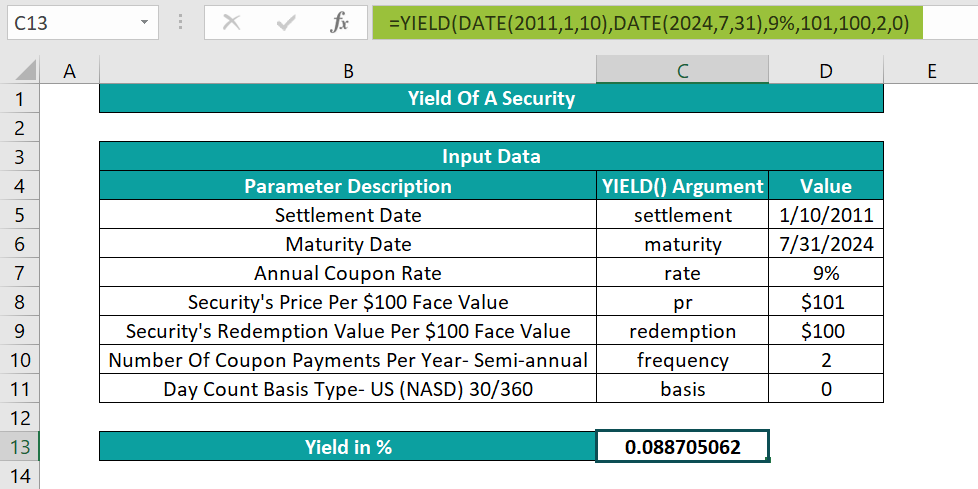Alternatively, we can apply the YIELD() from the Formulas tab by selecting the target cell C13 and clicking FormulasFinancialYIELD. This step will open the Function Arguments window.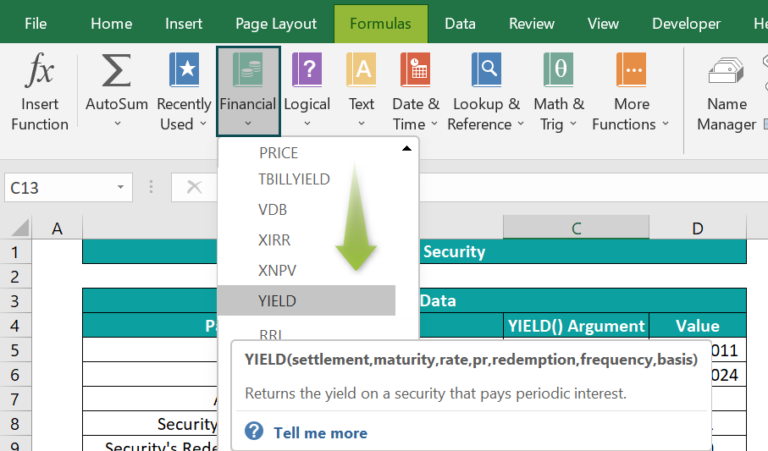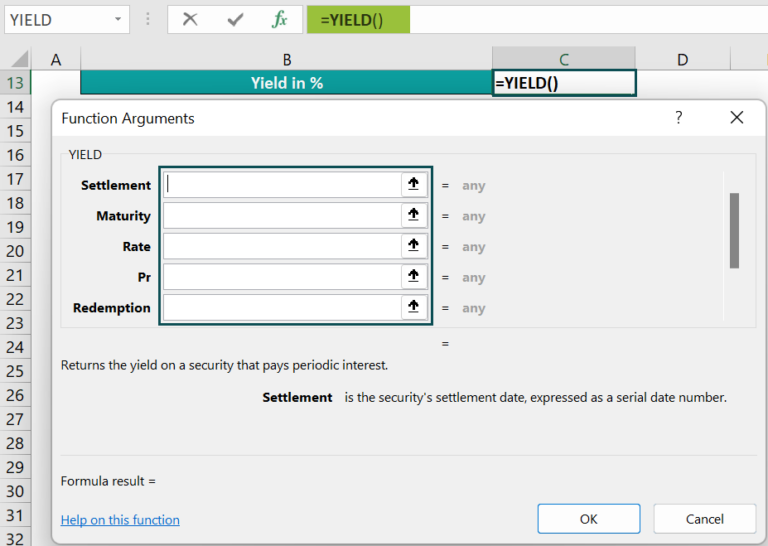Next, we must enter the YIELD() arguments in the Function Arguments window.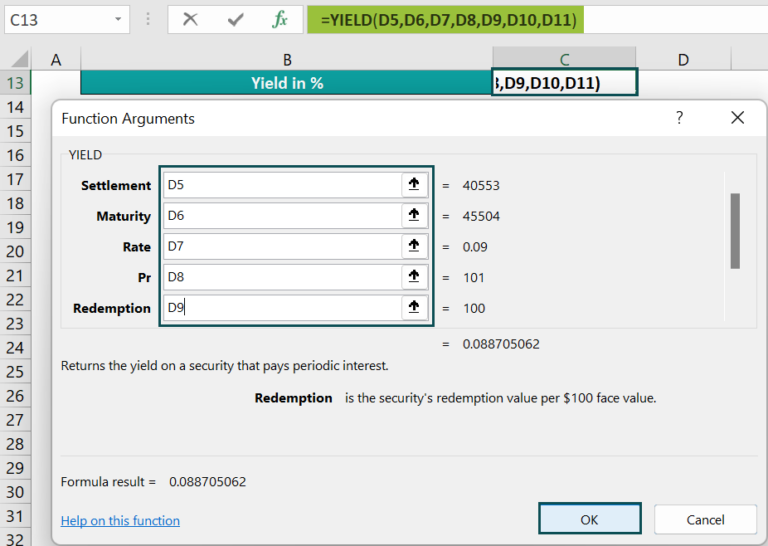And once we click OK in the Function Arguments window, the YIELD() gets executed in the target cell C13.

3. Select cell C13 and set the data format as Percentage using the Number Format option in the Home tab.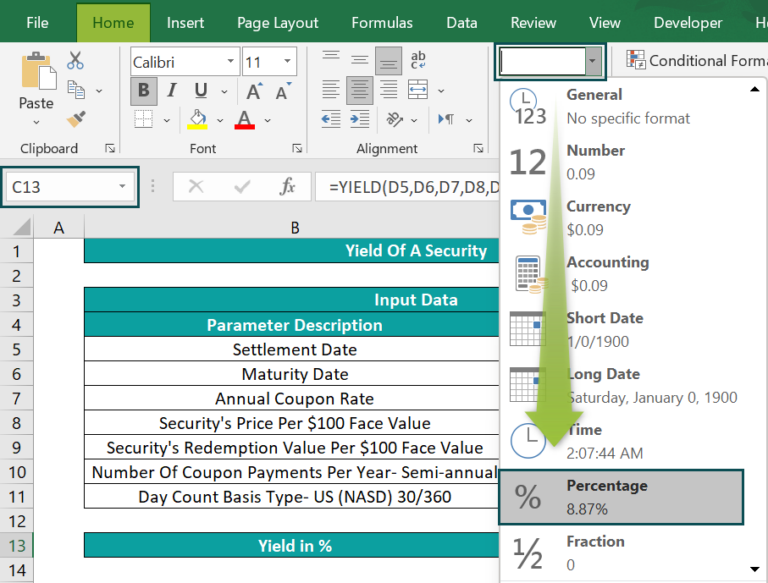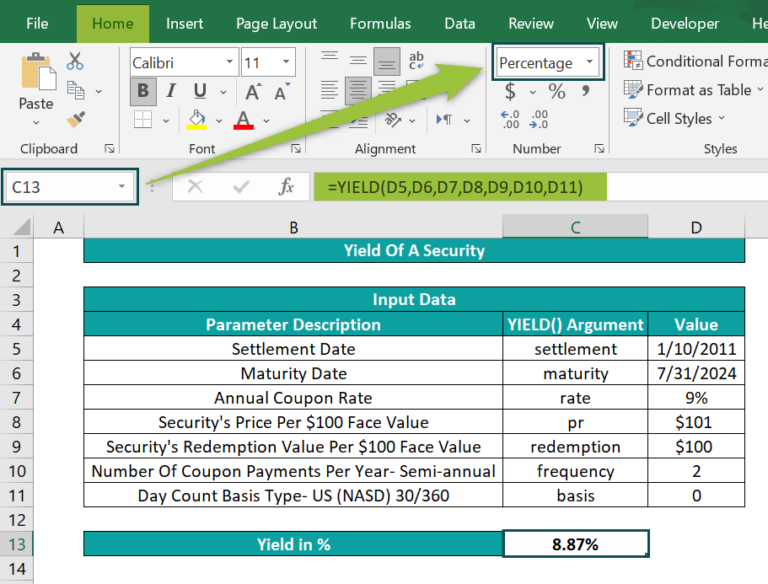Thus, we get the required yield of the specific security scheme as a percentage value of 8.87%.

### Examples

This section will show a few more examples of the Excel YIELD function.

#### Example #1

This example explains how to utilize the Excel YIELD function to determine the yield of a ten-year bond.

The table below contains the inputs required to calculate the ten-year bond yield.

Consider the specific bond’s issue date was 1/1/2010, and we purchased it six months later. So, the settlement date is 7/1/2010, and the maturity date is 1/1/2020. Also, assume the coupon payment frequency is annual and the day count basis is the default type.

And suppose we must display the resulting yield value in cell B13. Then we can use Excel YIELD function in the target cell to get the required outcome.

• Step 1: Select the target cell B13, enter the YIELD() provided in the Formula Bar in the below image, and press Enter.
• Step 2: Select cell B13 and set its data format as Percentage using the Number Format option in the Home tab.

Thus, for the given input values, the YIELD() returns the value 0.108466. And once we set the target cell data format as Percentage, you get the ten-year bond yield, 10.85%.

#### Example #2

Let us see the process of calculating a security’s yield with a quarterly coupon payment frequency using Excel YIELD function.

The following table contains the inputs required to find the yield of a security a person purchased on 3/15/2021, with an annual interest rate of 7%.

And suppose the requirement is to display the calculated yield value in cell B13. Then applying the Excel YIELD function in the target cell can fetch the required data.

• Step 1: Select the target cell B13, enter the YIELD() provided in the Formula Bar in the below image, and press Enter.
• Step 2: Select cell B13 and set its data format as Percentage using the Number Format option in the Home tab.

In this example, the coupon payment frequency is quarterly. And thus, the frequency argument value is 4. Also, the argument basis takes the default value, 0.

And thus, based on the given input, the yield of the specific security is 6.86%.

#### Example #3

Let us see the different scenarios when the Excel YIELD function returns error values.

Suppose we need to enter the YIELD() argument values in the table below, calculate the specific security yield, and display the output in cell B13.

So, we will enter the YIELD(),with the required inputs supplied, in the target cell B13. However, the Excel YIELD function might throw errors if the source data contains incorrect values.

• settlement or maturity – Invalid Date

Here, the maturity date is invalid; it is 11/31/2024 instead of 11/30/2024, leading to the YIELD() returning the #VALUE! error.

• YIELD() Argument Values – Non-numeric

In this case, the basis argument value should be 0, but the supplied value is “NIL”, which makes the YIELD() throw the #VALUE! error.

• settlement Greater than or Equal To maturity

The above data shows that the settlement date falls after maturity, causing the YIELD() to return the #NUM! error.

• rate Is Less Than 0

In this case, the rate argument value is a negative percentage value, leading to the YIELD() throwing an error.

• pr or redemption Is Less or Equal To 0

Here, the YIELD() output is the #NUM! error as the pr argument value is 0 instead of a value greater than zero.

• frequency Is Not 1, 2, or 4

The frequency argument value can be 1, 2, or 4. However, as per the given input, the argument frequency is 3, and thus the YIELD() output is an error value.

• basis Is Not 0, 1, 2, 3, or 4

The supplied basis argument value is 6, which is an incorrect value. The argument basis can be a value from 0 to 4.

Thus, the YIELD() will return the required value when we resolve the above issues.

### Important Things To Note

• It is best to supply the date arguments to the Excel YIELD function as cell references to dates or formulas returning dates. And if entering the date values directly in the YIELD(), use the DATE() to avoid supplying invalid date values.
• For invalid settlement or maturity dates or non-numeric argument values, the YIELD() throws the #VALUE! error.
• Suppose settlement is greater or equal to maturity, the rate is less than 0, pr or redemption <= 0, frequency is not 1, 2, or 4, or basis is not 0, 1, 2, 3, or 4. Then the YIELD() return value will be the #NUM! error.

1. Where is the YIELD function in Excel?

The YIELD function in Excel is in the Formulas tab. We can select FormulasFinancialYIELD to access the function.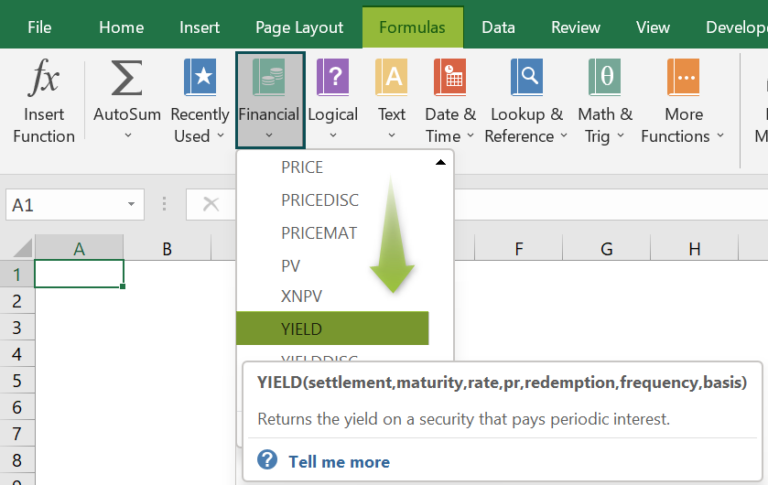2. What is the settlement in the YIELD function in Excel?

The settlement in the YIELD function in Excel indicates the date a buyer trades a coupon, such as a security or bond. For example, a 10-year bond issue date is 1/1/2010, and a buyer purchases it four months later. Then the settlement date will be 5/1/2010.

3. How can you determine the yield of a security with a quarterly coupon payment frequency and Actual/actual basis type using the Excel YIELD function?

We can determine the yield of a security with a quarterly coupon payment frequency and Actual/actual basis type using the Excel YIELD function in the following way.

Let us see the steps with an example.

The below table contains the required input for calculating the yield of a specific security.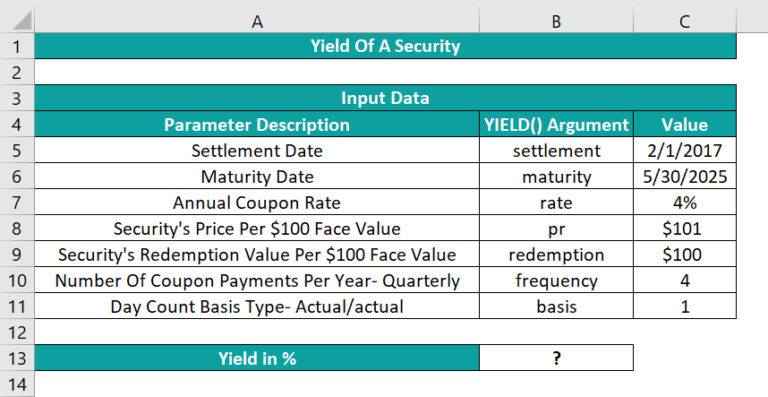In this example, the coupon payment frequency is quarterly. So, the frequency argument value is 4. And the day count basis type is Actual/actual. Hence, the basis argument value is 1.

Suppose the requirement is to show the calculated yield value in cell B13. Then, here is how we can apply the Excel YIELD function in the target cell and get the yield value.

• Step 1: Select the target cell B13, enter the YIELD() provided in the Formula Bar in the below image, and press Enter.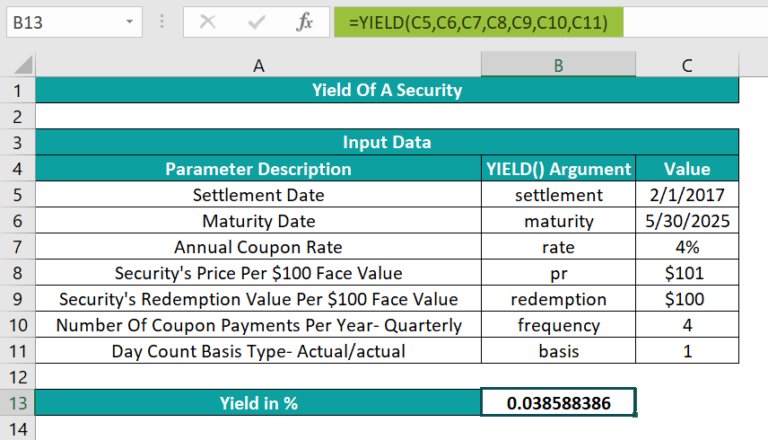• Step 2: Select cell B13 and set the data format as Percentage using the Number Format option in the Home tab.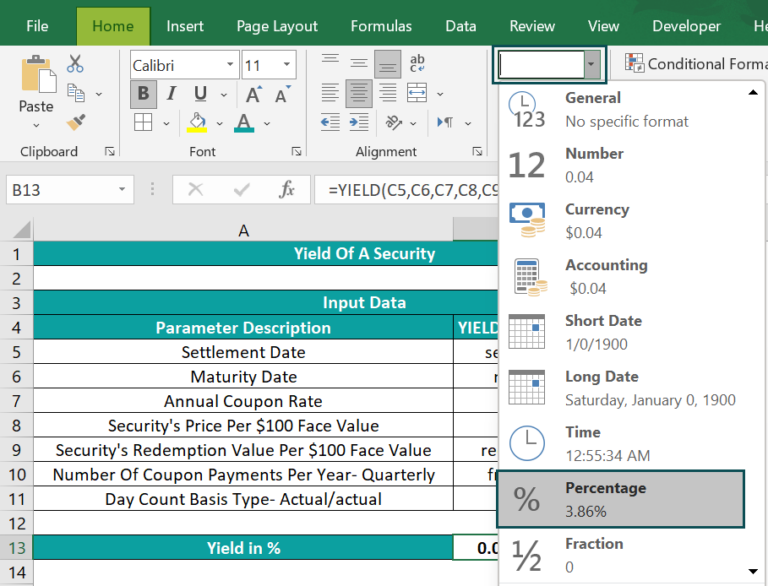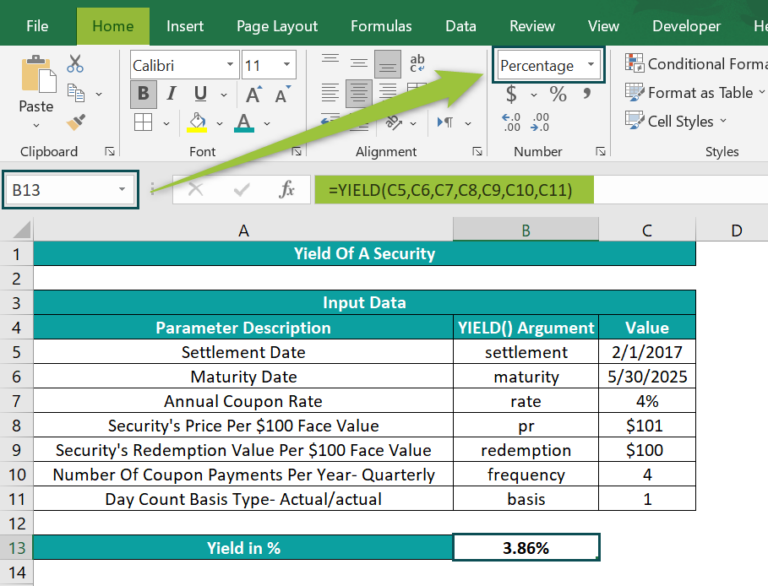Thus, the YIELD() evaluates the yield of a security, with quarterly coupon payment frequency and Actual/actual day count basis type, as 3.86%.